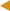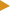﻿ FieldSet.Set Method : OnixS .NET FIX EngineRemove Method Table of Content Set Method (Int32, ArraySegment(Byte))FieldSet.Set MethodOverload List
NameDescriptionSet(Int32, ArraySegment<Byte>)
Sets the field value as a byte array from the array segment. Transforms input value into text-presentation, so actually stored value is text-presentation of input.Set(Int32, Boolean)
Sets the field value as Boolean. Transforms input value into text-presentation, so actually stored value is text-presentation of input.Set(Int32, Byte[])
Sets the field value as a byte array. Transforms input value into text-presentation, so actually stored value is text-presentation of input.Set(Int32, Char)
Sets the field value as a character. Transforms input value into text-presentation, so actually stored value is text-presentation of input.Set(Int32, DateTime)
Sets the field value as a DateTime object. Transforms input value into text-presentation, so actually stored value is text-presentation of input.Set(Int32, Decimal)
Sets the field value as a Decimal number. Transforms input value into text-presentation, so actually stored value is text-presentation of input.Set(Int32, Double)
Sets the field value as a double-precision floating point number. Transforms input value into text-presentation, so actually stored value is text-presentation of input.Set(Int32, Int32)
Sets the field value as an integer. Transforms input value into text-presentation, so actually stored value is text-presentation of input.Set(Int32, Int64)
Sets the field value as a signed 64-bit (8-byte) integer. Transforms input value into text-presentation, so actually stored value is text-presentation of input.Set(Int32, String)
Sets the field value as string.Set(Int32, StringBuilder)
Sets the field value from the given StringBuilder instance.Set(Int32, TimeSpan)
Sets the field value as a TimeSpan object. Transforms input value into text-presentation, so actually stored value is text-presentation of input.Set(Int32, HighResolutionTimeSpan, TimeSpanFormat)
Sets the field value as a HighResolutionTimeSpan object. Transforms input value into text-presentation with the given format, so actually stored value is text-presentation of input.Set(Int32, HighResolutionTimestamp, TimestampFormat)
Sets the field value as a HighResolutionTimestamp object. Transforms input value into text-presentation with the given format, so actually stored value is text-presentation of input.Set(Int32, DateTime, TimestampFormat)
Sets the field value as a DateTime object. Transforms input value into text-presentation with the given format, so actually stored value is text-presentation of input.Set(Int32, Decimal, Int32)
Sets the field value as a Decimal number. Transforms input value into text-presentation, so actually stored value is text-presentation of input.Set(Int32, Double, Int32)
Sets the field value as a double-precision floating point number. Transforms input value into text-presentation, so actually stored value is text-presentation of input.Set(Int32, TimeSpan, TimeSpanFormat)
Sets the field value as a TimeSpan object. Transforms input value into text-presentation with the given format, so actually stored value is text-presentation of input.
TopSee Also